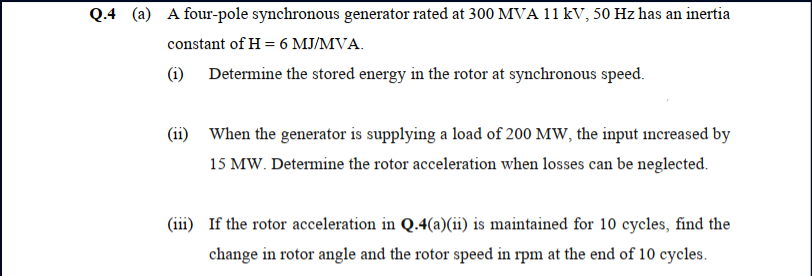# (a) A four-pole synchronous generator rated at 300 MVA 11 kV, 50 Hz has an inertia constant of H = 6 MJ/MVA. (1) Determine the stored energy in the rotor at synchronous speed. (ii) When the generator is supplying a load of 200 MW, the input increased by 15 MW. Determine the rotor acceleration when losses can be neglected. (iii) If the rotor acceleration in Q.4(a)(ii) is maintained for 10 cycles, find the change in rotor angle and the rotor speed in rpm at the end of 10 cycles.

Questionhelp_outlineImage Transcriptionclose(a) A four-pole synchronous generator rated at 300 MVA 11 kV, 50 Hz has an inertia constant of H = 6 MJ/MVA. (1) Determine the stored energy in the rotor at synchronous speed. (ii) When the generator is supplying a load of 200 MW, the input increased by 15 MW. Determine the rotor acceleration when losses can be neglected. (iii) If the rotor acceleration in Q.4(a)(ii) is maintained for 10 cycles, find the change in rotor angle and the rotor speed in rpm at the end of 10 cycles. fullscreen

### Want to see this answer and more?

Experts are waiting 24/7 to provide step-by-step solutions in as fast as 30 minutes!*

*Response times may vary by subject and question complexity. Median response time is 34 minutes for paid subscribers and may be longer for promotional offers.
Tagged in
Engineering
Electrical Engineering

### Power Systems